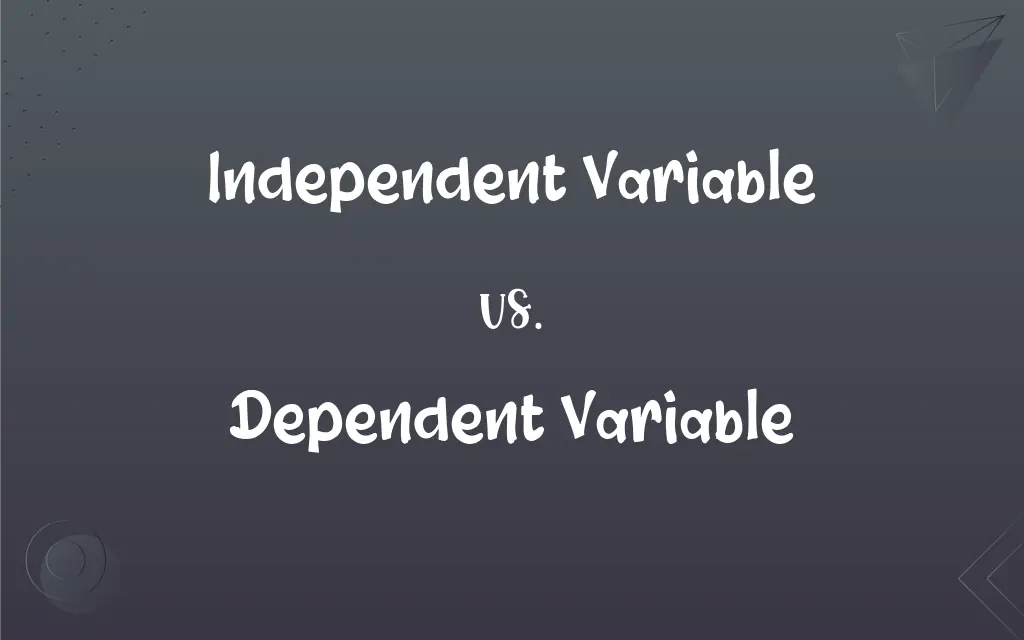# Independent Variable vs. Dependent Variable: What's the Difference?Edited by Huma Saeed || By Sumera Saeed || Published on November 18, 2023
The independent variable is manipulated to observe its effect, while the dependent variable is the outcome or response being measured.## Key Differences

In experiments, the independent variable is what researchers alter or change to examine its influence. The dependent variable then is the observed outcome or the response that might change due to this manipulation.
When examining cause and effect, the independent variable is viewed as the potential cause. In contrast, the dependent variable reflects the effect or result of that particular cause.
Graphically, in most contexts, the independent variable is plotted on the x-axis, representing the input. The dependent variable is usually on the y-axis, showcasing the outcome.
In terms of control, researchers have direct control over the independent variable as they decide its levels or categories. However, they only observe or measure the dependent variable without directly manipulating it.
Both the independent variable and dependent variable are crucial components of experimental research, ensuring that studies can test hypotheses and draw valid conclusions.

## Comparison Chart

### Role in Experiment

Manipulated or altered by researchers.
Outcome or response being measured.

### Relation to Cause & Effect

Considered the cause.
Reflects the effect or result.

### Graphical Representation

Typically plotted on the x-axis.
Typically plotted on the y-axis.

### Control Level

Directly controlled by researchers.
Only observed or measured, not manipulated.

### Purpose in Research

To examine its influence on an outcome.
To observe changes due to the independent variable.

## Independent Variable and Dependent Variable Definitions

#### Independent Variable

The presumed cause in a cause-and-effect relationship.
In researching the impact of study hours on exam scores, the study hours are the independent variable.

#### Dependent Variable

The result that might change due to the independent variable.
When analyzing study hours' impact on exam scores, the scores are the dependent variable.

#### Independent Variable

The factor that is varied in experimental research.
In analyzing the effect of temperature on solubility, temperature is the independent variable.

#### Dependent Variable

The observed outcome or response in an experiment.
In a study on light affecting plant growth, the plant's height is the dependent variable.

#### Independent Variable

The variable representing categories or levels in a study.
In an experiment on drug efficacy, drug dosage is the independent variable.

#### Dependent Variable

The variable that captures the potential effects of the independent variable.
In an experiment on drug efficacy, the relief of symptoms is the dependent variable.

#### Independent Variable

The variable that is deliberately changed in an experiment.
In a study on light affecting plant growth, the amount of light is the independent variable.

#### Dependent Variable

The output variable in experimental research.
In testing different diets for weight loss, the weight lost is the dependent variable.

#### Independent Variable

The input variable that researchers manipulate.
In testing different diets for weight loss, the type of diet is the independent variable.

#### Dependent Variable

The factor that researchers measure without manipulating.
In a study on temperature's effect on solubility, the amount dissolved is the dependent variable.

## FAQs

#### What does the independent variable represent in an experiment?

The independent variable represents the factor being manipulated or changed.

#### Can there be multiple independent variables in a study?

Yes, studies can have multiple independent variables; these are called multivariate studies.

#### Is the dependent variable the outcome of an experiment?

Yes, the dependent variable is the observed outcome or response.

#### Which variable is plotted on the x-axis of a graph?

Typically, the independent variable is plotted on the x-axis.

#### Do researchers directly manipulate the dependent variable?

No, researchers observe or measure the dependent variable but don't directly manipulate it.

#### Why is it essential to identify the independent variable in research?

Identifying the independent variable is crucial to establish cause-and-effect relationships.

#### Does the independent variable always cause a change in the dependent variable?

Not necessarily. Sometimes, changing the independent variable doesn't affect the dependent variable.

#### How do researchers ensure that only the independent variable affects the dependent variable?

By controlling other variables, called control variables, to isolate the independent variable's effect.

#### How do independent and dependent variables relate to control variables?

While the independent variable is manipulated and the dependent variable is observed, control variables are kept constant to ensure they don't influence the dependent variable.

#### How does the dependent variable change?

The dependent variable may change in response to alterations in the independent variable.

#### Is the dependent variable the effect in a study?

Yes, the dependent variable reflects the effect or result of the independent variable.

#### Can the roles of variables switch in different experiments?

Yes, in different studies, what was once an independent variable can become a dependent variable, and vice versa.

#### Can the same variable be both independent and dependent in different contexts?

Yes, depending on the research question, a variable's role can change.

#### How is the independent variable chosen?

Researchers choose the independent variable based on their hypothesis or research question.

#### Can the dependent variable be predicted using the independent variable?

Often, researchers aim to predict the dependent variable based on changes in the independent variable.

#### Why is the dependent variable essential in research?

The dependent variable allows researchers to assess the effect of changes in the independent variable.

#### In which variable do researchers expect to see changes during an experiment?

Researchers expect to see changes in the dependent variable based on manipulations of the independent variable.

#### Why are both independent and dependent variables vital for experimental validity?

Both are crucial for testing hypotheses and drawing valid conclusions about cause-and-effect relationships.

#### Can the dependent variable be controlled?

No, the dependent variable is observed and measured, not controlled.

#### Is the dependent variable the same as the response variable?

Yes, in many contexts, the dependent variable is also termed the response variable.